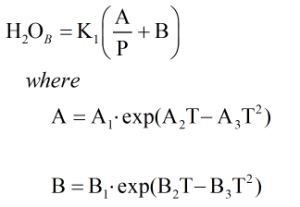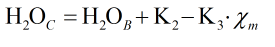# C300 – Water Content in Natural Gas – Bukacek Method

## Description

This calculates the water content in natural gas with the option to correct for methanol.

Equilibrium Moisture Content of Natural Gases – Institute of Gas Technology – R.F Bukacek

Kelton calculation reference C300

FLOCALC calculation reference F100

## Options

### Correction for Methanol

• Apply

This option is chosen to correct for methanol when carrying out the calculation.

## Calculation

### Water Content

The water content is calculated by:Where P = Pressure T = Temperature Ax = Constant Bx = Constant K1 = Constant

### Correction for Methanol

If a correction for methanol is made water content is calculated byWhere χm = methanol content K2 = Constant K3 = Constant

Back